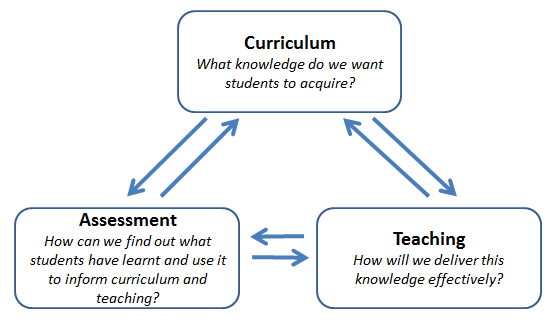top of page
Search

# Assessment for, as and of learning in MathFor over 30 years, I have been teaching high school math with a goal in mind of

keeping it fun and rigorous. As a student, I found that I learned math differently than my teachers wanted. My brain saw the path to the solution differently than the book but I still got the same answer. This was an issue for my teachers because they didn't always get how I saw my way to the answer.

So, I used this view of education for my classes. I have eliminated all tests and quizzes in my classes. We instead do a unit ending check-in. this is an open book, calculators and desmos allowed assignment. I have developed questions that have several steps for solving. Each question is out of 5 marks. 4 marks are for the logical process show to get to the answer and 1 mark for the correct answer. I cannot in good conscience give a 0 for the wring answer if my students have shown a logical solution but made a minor mistake. Math is not an all or nothing program.

Mathematics should be built on the understanding of the process of math and not just the answers. My students are always trying to see how their brain understands the math. They need to figure out how to get to the solution and not just an answer.

How can we find out what students have learnt and use it develop deeper understanding?

For me, math learning develops throughout the year. We are not emphasizing the snapshot of what they learn. I need to understand where they are in the understanding and what we need to work on to move forward in the complexities of math. That is the main reason I removed testing. With the check in process, they can continue to correct the work and show me how the knowledge has changed or grown with each attempt at the long questions. My students are always working on evolving their math understanding and we can take steps forward or go back to the beginning topics at any point and time. If we need to revisit the beginning stages of math, we can or we can jump to an extremely complicated topic. It all depends on where the students understanding is at that time and throughout the year.

I find the fluidity of my math classes to be beneficial to my students greater understanding of math and its great beauty. If you are looking for your students to develop that greater understanding, then you should drop the old test ways and develop a fun and energetic math classroom where the whole math student is more than the snapshot test kid.